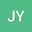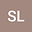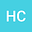Existence and multiple solutions for the critical fractional $p$-Kirchhoff type problems involving sign-changing weight functions
•••Senli Liu
Central South University School of Mathematics and Statistics
Author ProfileHaibo Chen
Department of Mathematics, Central South University
Author Profile## Abstract

The aim of this paper is to study the existence and multiplicity of nonnegative solutions for the following critical Kirchhoff equation involving the fractional $$p$$-Laplace operator $$(-\Delta)_{p}^{s}$$. More precisely, we consider

\begin{equation} \begin{cases}M\left(\iint_{\mathbb{R}^{2N}}\frac{|u(x)-u(y)|^{p}}{|x-y|^{N+ps}}dxdy\right)(-\Delta)_{p}^{s}u=\lambda f(x)|u|^{q-2}u+K(x)|u|^{p_{s}^{*}-2}u,&{\rm in}\ \Omega,\\ u=0,&{\rm in}\ \mathbb{R}^{N}\setminus\Omega,\\ \end{cases}\nonumber \\ \end{equation}

where $$\Omega\subset\mathbb{R}^{N}$$ is an open bounded domain with Lipschitz boundary $$\partial\Omega$$, $$M(t)=a+bt^{m-1}$$ with $$m>1,a>0,b>0$$, dimension $$N>sp$$, $$p_{s}^{*}=\frac{Np}{N-ps}$$ is the fractional critical Sobolev exponent, and the parameters $$\lambda>0,0<s<1<q<p<\infty$$. Applying Nehari manifold, fibering maps and Krasnoselskii genus theory, we investigate the existence and multiplicity of nonnegative solutions.

01 Mar 2020Submitted to Mathematical Methods in the Applied Sciences
07 Mar 2020Submission Checks Completed
07 Mar 2020Assigned to Editor
10 Jul 2020Reviewer(s) Assigned
19 Oct 2021Editorial Decision: Revise Minor